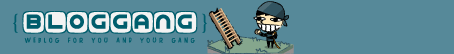Group Blog
 Group ตัวอย่าง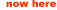<< กุมภาพันธ์ 2551
 1 2 3 4 5 6 7 8 9 10 11 12 13 14 15 16 17 18 19 20 21 22 23 24 25 26 27 28 29

 19 กุมภาพันธ์ 2551
 All Blogs
FTE Calculation

HOW TO CALCULATE FTES
DEFINITIONS:
FTE stands for full time equivalent.
An FTE is the equivalent of one person working full time:
8 hrs./day
X 5 days/week
X 52 weeks/year
= 2080 hours/year
Examples:
 1 person working full time = 1 FTE
 2 people each working 4 hours per day, 5 days per week = 1 FTE
TIPS:
 FTEs does NOT mean number of employees.
 An FTE value stays the same over time (except when you adjust your usual staffing pattern). Do not multiply it by days, weeks, or months.
HOW TO DO IT:
1. If you know your total number of labor hours for one year: Divide by 2080. This is your FTEs.
Example:Your department's total labor hours as reported to payroll for Jan. - Dec. 1998 were 13,104.

13,104 ÷ 2080 = 6.3 FTEs.
ALTERNATE METHOD:
If you know your total number of labor hours for one month: Divide by 173.33. This is your FTEs.
Example: Your department's total labor hours as reported to payroll for May 1999 were 3,600.

3,600 ÷ 173.33 = 20.77 FTEs.
ALTERNATE METHOD:
Calculate the number of labor hours you require in one day. Divide by 8. This is your FTEs.
Example: Your know your daily staffing uses 9 people working 8 hours, plus 1 person working 3 hours. You use some full-time and some part-time employees each day to achieve this.

Your daily staffing = (9 people X 8 hrs.) + (1 person X 3 hrs.) = 75 people hours.

75 ÷ 8 = 9.375 FTEs
Calculating FTE (Full Time Equivalent) for Salary and Annual Leave

1. Part time staff working 52 weeks

Number of hours worked
37 Hours

For example an employee working 18.5 hours per week for 52 weeks the FTE would be calculated as follows:-

18.5 = 0.5 FTE
37

0.5 x Full time salary = Pro rata salary

0.5 x Full time annual leave entitlement = Pro rata annual leave

Pro rata annual leave may be calculated in days or hours, one days annual leave for a full time member of staff is equivalent to 7 hours and 24 minutes.

2. Salary and Annual Leave Payments for Part Year Staff (Less than 52 weeks)

Part year staff are paid a twelfth of their annual salary on a monthly basis. Annual leave is deemed to be taken during scheduled non-working weeks. Payment for annual leave is included in the annual salary and is calculated as follows:-

No. of weeks worked x Annual Holidays = Number of days annual
Number of weeks worked by a full time employee leave Entitlement

No. of weeks worked x Bank and Concessionary Holidays = No. of Bank and
Number of weeks worked by a full time employee Concessionary
Holidays

Annual Leave + Bank and Concessionary Days = Number of Additional
5 (days per week) Weeks Paid

3. Calculating an FTE for Part time staff working less than 52 weeks

The additional weeks paid are included within the FTE calculation for part time staff working less than 52 weeks. Annual leave (including bank and concessionary holidays) for full time employees are as follows:-

Annual Leave Entitlement = 25 days (For employees with less than 1 years service at UWIC)

Bank and Concessionary Holidays = 12 days

Total Annual Leave, Bank and Concessionary Holiday = 37 days ÷ 5 (days per week) = 7.4 weeks

Therefore a full time member of staff actually works

52 weeks  7.4 weeks = 44.6 weeks

To calculate the number of weeks paid the following formula is used:-

Number of weeks worked
44.6 Weeks

To calculate an FTE this then needs to be multiplied by the following formula:-

Number of hours worked
37 Hours

For example an employee working 36 weeks per year and 18.5 hours per week with an annual leave entitlement of 25 days the FTE would be calculated as follows:-

36 x 18.5 = 0.40 FTE
44.6 37

0.4 x Full time salary = Pro rata salary
3. Calculating an FTE for Part time staff working less than 52 weeks

The additional weeks paid are included within the FTE calculation for part time staff working less than 52 weeks. Annual leave (including bank and concessionary holidays) for full time employees are as follows:-

Annual Leave Entitlement = 25 days (For employees with less than 1 years service at UWIC)

Bank and Concessionary Holidays = 12 days

Total Annual Leave, Bank and Concessionary Holiday = 37 days ÷ 5 (days per week) = 7.4 weeks

Therefore a full time member of staff actually works

52 weeks  7.4 weeks = 44.6 weeks

To calculate the number of weeks paid the following formula is used:-

Number of weeks worked
44.6 Weeks

To calculate an FTE this then needs to be multiplied by the following formula:-

Number of hours worked
37 Hours

For example an employee working 36 weeks per year and 18.5 hours per week with an annual leave entitlement of 25 days the FTE would be calculated as follows:-

36 x 18.5 = 0.40 FTE
44.6 37

0.4 x Full time salary = Pro rata salary

Annual Leave Entitlement = 35 days

Bank and Concessionary Holidays = 12 days

Total Annual Leave, Bank and Concessionary Holiday = 47 days ÷ 5 days = 9.4 weeks

Therefore a full time member of staff actually works

52 weeks  9.4 weeks = 42.6 weeks

To calculate the number of weeks paid the following formula is used:-

Number of weeks worked
42.6 Weeks

To calculate an FTE this then needs to be multiplied by the following formula:-

Number of hours worked
37 Hours

For example an employee working 36 weeks per year and 18.5 hours per week with an annual leave entitlement of 35 days the FTE would be calculated as follows:-

36 x 18.5 = 0.42 FTE
42.6 37

0.42 x Full time salary = Pro rata salary

Create Date : 19 กุมภาพันธ์ 2551
Last Update : 19 กุมภาพันธ์ 2551 17:49:39 น. 0 comments
Counter : 1271 Pageviews.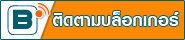ชื่อ : * blog นี้ comment ได้เฉพาะสมาชิก
Comment :
*ส่วน comment ไม่สามารถใช้ javascript และ style sheet

 powerploy
Location :

[ดู Profile ทั้งหมด]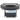ให้ทิปเจ้าของ Blog [?]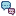ฝากข้อความหลังไมค์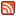Rss Feed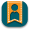ผู้ติดตามบล็อก : 1 คน [?]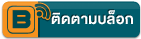Friends' blogs